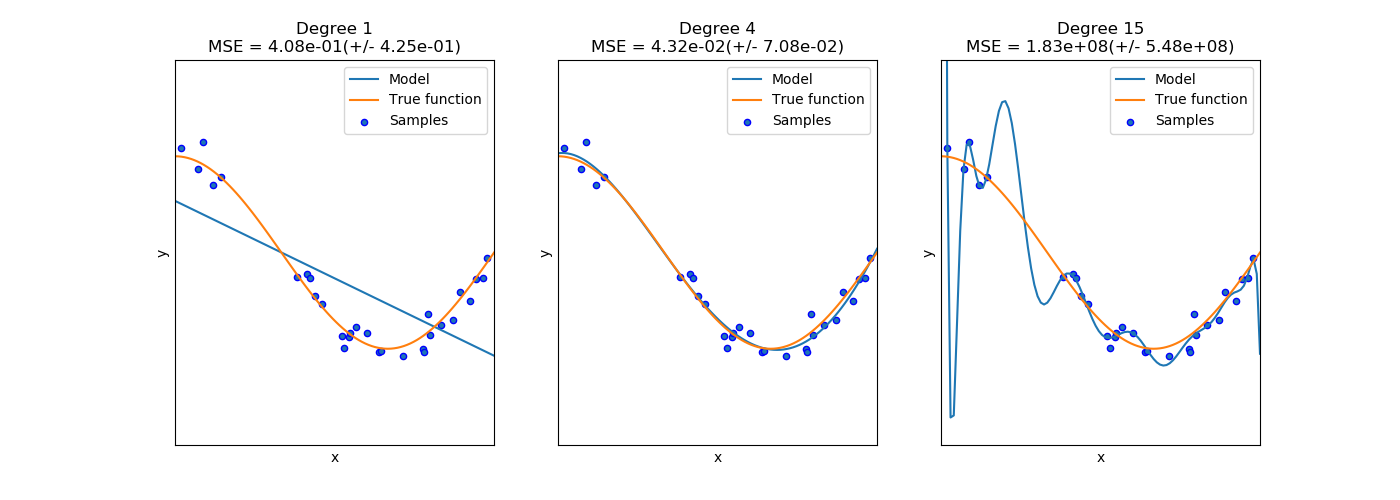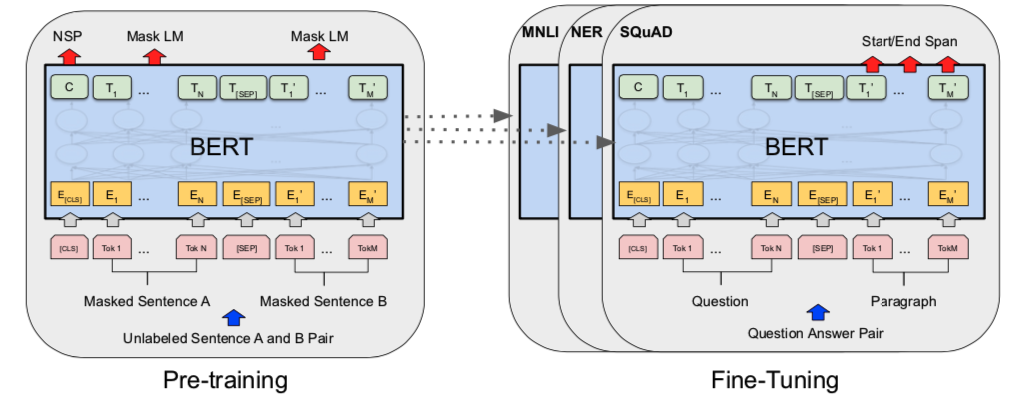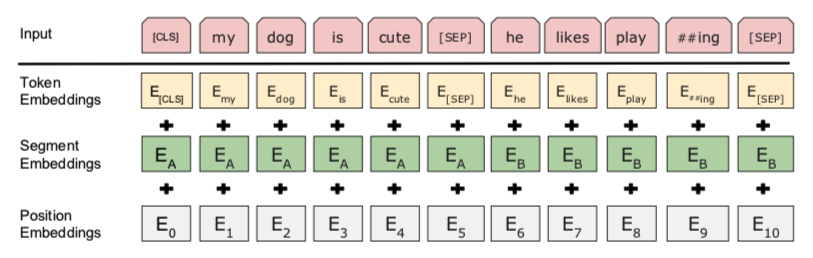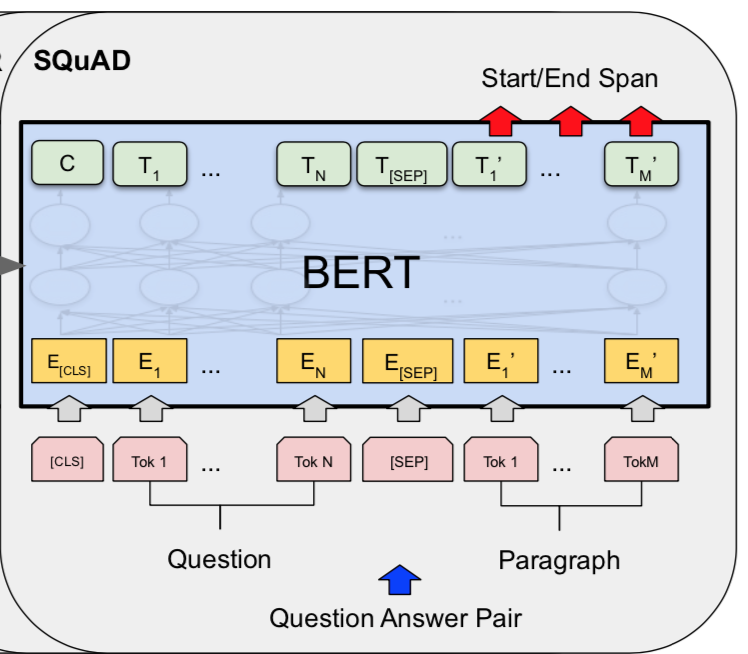## 8.1 Supervised learning

Supervised learning is the most widely used ML type in practice. The task of supervised learning is to learn how to map an input to an output, for example the picture of a cat to the category of “cat”

Supervised learning basics:• Supply model with
• input
• image of cat
• output
• label “cat”
• Learn function to map input to output
• $$outcome = f(features) = f(X_1, X_2, \dots, Xp) = f(X)$$
• Two main applications
• classification
• regression
• Main algorithms
• linear regression see chapter 9.1
• logistic regression see chapter 9.2
• tree based methods see chapter 9.3
• support vector machines see chapter 9.4
• neural networks see chapter 9.5

Choosing input representation and model are crucial for prediction performance.

Choosing input representation and model:

• Finding suitable input see chapter on feature engineering 7.4
• Finding suitable model see chapter on model fit 7.5
• bias variance dilemma20
• trade bias and variance (see image below)
• overfitting
• to complex model for given problem or data (see image below)
• more data
• or less complex model### 8.1.1 Self supervised learning

Training a neural network from scratch, i.e. with random weights can be very computer intensive and might need a huge amount of data. One way to reduce the necessary amount of data is to use a pre-trained network. For computer vision and natural language processing there are plenty of pre-trained networks available (ULMFIt, GPT-2, VGG, GoogleNet, ResNet).

The task at hand can be different to what the task was when the network was trained. In self supervised learning the task worked on during training is called “pretext task”, the task which uses the pre-trained net is called “downstream task”. For the pretext task the model is trained using labels that are part of the input data, therefore no separate external labels are needed.. A practical overview on self supervised learning is given in a blog by FastAI https://www.fast.ai/2020/01/13/self_supervised/#consistency-loss

Self supervised learning:

• Pre-trained networks
• reduce training time
• reduce necessary data amount
• Pre-training
• no external labels needed
• Fine tuning
• less data
• faster

Example for self supervised learning: BERT

BERT stands for Bidirectional Encoder Representations from Transformers and is a language representation model, introduced in 2018 . BERT is designed to pre-train deep bidirectional representations from unlabeled text. It can be fine tuned with just one additional output layer. The model architecture is a multi-layer bidirectional Transformer encoder based on the original implementation described in . Background description can be found at as well as excellent guides such as “The Annotated Transformer” http://nlp.seas.harvard.edu/2018/04/03/attention.html

BERT facts:

• designed to pre-train deep bidirectional representations
• 3,300M words (Wikipedia and BooksCorpus)
• model architecture (see image below)
• multi-layer bidirectional Transformer encoder
• next sentence prediction
• add one more output layer
• choose most plausible continuation sentence out of four possibilities
• Complexity of model
• BERT base $$\implies$$ 110M parameters
• BERT large $$\implies$$ 340M parameters

The architecture of BERT is shown in image below.Figure from

Input/Output Representations

A sentence can be a single sentence or a pair of sentences. A “sequence” refers to the input token sequence to BERT, which may be a single sentence or two sentences packed together. The words are coded using WordPiece embeddings. The first token of every sequence is a special classification token (CLS). The final hidden state of CLS is used for classification tasks. Sentence pairs are in single sequence, separated by special token (SEP), and a learned embedding to every token indicating to which sentences it belongs, A or B.

The input embeddings are denoted “E,” the final hidden vector for CLS is C and the final hitten vector for the $$i_{th}$$ as $$T_{i}$$

Input/Output Representations:

• Token embedding
• WordPiece, 30,000 token vocabulary
• First token
• special token CLS
• its hidden state at the output used for classification
• Segment embedding
• indicated whether word belongs to sentence “A” or “B”
• Position embedding
• indicates the position of token in sequence
• input embeddings are sum of
• token embeddings
• segmentation embeddings
• position embeddings.

The input representation of BERT is shown below.Figure from

In the Stanford Question Answering Dataset (SQuAD v1.1) a question and a passage of Wikipedia with the answer is given. The task is to predict the answer text span in the passage. The question is represented as sentence “A,” the Wikipedia passage in sentence “B.” Based on the the hidden states $$T_i$$ and the vectors $$S$$ and $$E$$ the start and end of the answer span is determined.

• Input
• question
• answer with a passage of Wikipedia
• Output
• start and end position of answer in passageFigure from

The results achieved by BERT for SQuAD v1.1 is shown in image belowFigure from

### References

Devlin, Jacob, Ming-Wei Chang, Kenton Lee, and Kristina Toutanova. 2018. “Bert: Pre-Training of Deep Bidirectional Transformers for Language Understanding.” arXiv Preprint arXiv:1810.04805.
Vaswani, Ashish, Noam Shazeer, Niki Parmar, Jakob Uszkoreit, Llion Jones, Aidan N. Gomez, Lukasz Kaiser, and Illia Polosukhin. 2017. “Attention Is All You Need.” CoRR abs/1706.03762. http://arxiv.org/abs/1706.03762.

1. source Wikipedia: In statistics and machine learning, the bias–variance tradeoff is the property of a set of predictive models whereby models with a lower bias in parameter estimation have a higher variance of the parameter estimates across samples, and vice versa. The bias–variance dilemma or bias–variance problem is the conflict in trying to simultaneously minimize these two sources of error that prevent supervised learning algorithms from generalizing beyond their training set↩︎